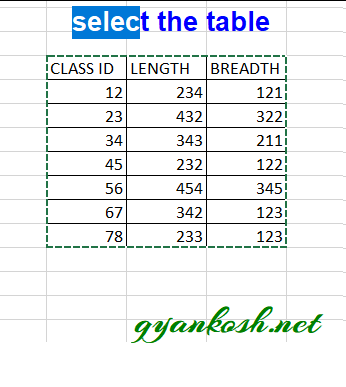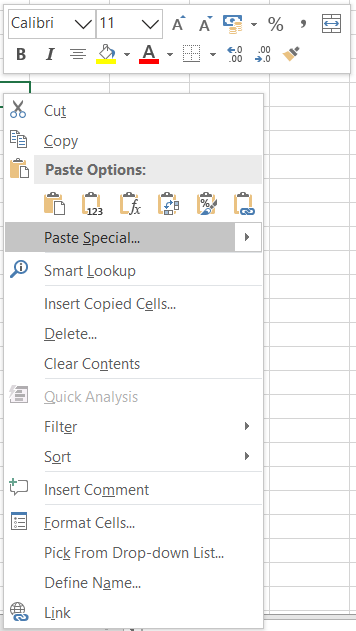PROVIDING ACCURATE, RELIABLE AND STRAIGHT TO THE POINT KNOWLEDGE ABOUT DIFFERENT IT TOOLS EXPLAINED WITH PRACTICAL EXAMPLES

# ROTATE A TABLE IN EXCEL

## INTRODUCTION

Suppose we have a data which needs to be rotated i.e. the rows should become the columns and vice versa. How would we do that?

One method is , to create a new sheet and start doing this manually but would that be an efficient solution. What if the report is too big like 1000 lines or so.

The article is about rotating a table i.e. Column becomes the rows and rows become the column or we can say rotate the table by 90 degrees.
This kind of need can emerge from any specific requirement or the layout issues. But whatever be the reason, we should always be ready for such situation and that is why we are going to discuss this issue here.
We’ll discuss the different ways of rotating a table in excel.
The solution to our problem is given by a function named Transpose which is a technical term for rotating any data.
We can perform this task in two ways mainly.
1. Using RIGHT CLICK SPECIAL PASTE menu.
2. Using TRANSPOSE FUNCTION

## ROTATING A TABLE USING SPECIAL PASTE MENU

We can rotate the table using the RIGHT CLICK SPECIAL PASTE Menu.
Before understanding the logics, let us perform the task first.
Here are the steps to rotate the table using Special Paste.
Suppose we have the following table.
 CLASS ID LENGTH BREADTH 12 234 121 23 432 322 34 343 211 45 232 122 56 454 345 67 342 123 78 233 123

STEPS:

• Select the complete table which you want to rotate including the headers.
•• Press CTRL+C or RIGHT CLICK> COPY.
• Select the cell where you want to paste the Rotated table and RIGHT CLICK. [ The first cell of the rotated table will be filled at the cell selected]
• GO TO PASTE   SPECIAL.• The following dialog box will open.

Click on the TRANSPOSE CHECKBOX on the lower right bottom of the dialog box.

• Click OK.
• The table will be properly pasted but the layout will be rotated.
• The output table is shown below.

## ROTATE TABLE USING TRANSPOSE FUNCTION IN EXCEL

The table can also be rotated using a function.

This can be done with the use of a function named as TRANSPOSE FUNCTION. [ CLICK HERE FOR FULL INFORMATION]

For a review the syntax ( the way how formula is phrased for excel) of MATCH is

=TRANSPOSE(ARRAY OF CELLS)

ARRAY ARRAY IS A GROUP OF CELLS WHICH CAN BE DECLARED AS A RANGE .
ARRAY  can be like A1:A10 OR A1:C10 with three columns and ten rows.
or
{1;2;3;4}

## STEPS TO ROTATE THE TABLE USING TRANSPOSE FUNCTION

### STEPS:

Here are the steps to rotate the table using transpose function.
Let us try to transpose the following table.
Suppose the following TABLE is present
 S.NO. COUNTRY CAPITAL 1 INDIA DELHI 2 UK LONDON 3 FRANCE PARIS 4 US WASHINGTON 5 INDONESIA JAKARTA
We will transpose this array using the TRANSPOSE function.

### STEPS:

Follow the following steps to rotate the table in EXCEL.
REFER PICTURE: ABOVE
1. Check the number of rows and columns of the given table. e.g. our example table has 3 columns and 6 rows. Now select the layout for the transposed table i.e. 3 rows and 6 columns. Now do nothing, and start typing the formula starting with  an “=”. The selection won’t be removed.
2. Now type the formula =TRANSPOSE(C3:E8) but don’t PRESS ENTER.
3. Press CTRL+SHIFT+ENTER.
4. The selected table will be rotated [transposed]

• ## WHAT IS EXCEL, HOW EXCEL WORKS, WRITE FORMULA IN EXCEL, EXAMPLE OF USING FORMULA IN EXCEL, ANIMATED EXAMLPE OF USING FORMULA IN EXCEL

LEARN

• EXCEL
• JAVASCRIPT
• MORE TO COME…

Encourage us by following at …

If you have any queries or suggestions or any problem which you would like us to solve for you, kindly mail at

[email protected]